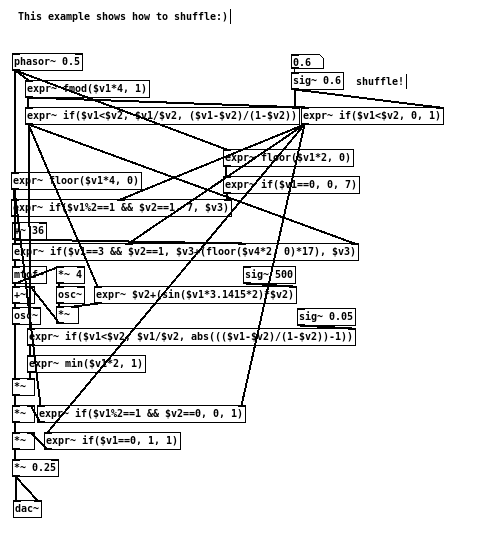```                  ______
/      |       ________
/       |____  /        \
/        |    \/   _      \
\       ___       /_\      \
\      \_/          .      \
\          /\_____/       /
\________/      |       /
|______/

```

# [THE_EXPR_METHOD] 010 Shuffle

20160422

When creating subdivisions of time with fmod() and floor(), it would produce equal segments by default. This is often convenient for further processing, because the duration of each measures are identical. However, it may not always be so musically desirable to derive time intervals in such a uniformed manor. Often, a slight shuffle is intentionally introduced into repeating rhythmic patterns, so to prevent the end result from sounding too static. This is generally referred to as the “swing”.

To shuffle a sawtooth signal, the following method can be used:

```   [phasor~ 0.5]                                 [sig~ 0.6]
|                                             |
[expr~ if(\$v1<\$v2, \$v1/\$v2, (\$v1-\$v2)/(1-\$v2))]
|
[s~ shuffled_signal]
```

The second inlet(\$v2) of the [expr~] signifies the amount, as well as the direction, of the shuffle. When set to 0.5, the input phasor would be divided equally, where as 0.6 would increase the duration of the first section, making it a little be longer than the second segment. Note that the allowed value for \$v2, in this case, would be between 0 and 1.

Once a sawtooth signal can be halved in arbitrary proportion, it would also be useful to be able to identify the current position of the signal, in relation to the two uneven portions of the “swing”.

To to this, the following can be used in combination with the previous example.

```   [phasor~ 0.5]           [sig~ 0.6]
|                       |
[expr~ if(\$v1<\$v2, 0, 1)]
|
[s~ shuffle_idx]
```

In this case, the two sections can now be identified with an index value of 0 and 1. It is worth noting that, with shuffled signal, it would be more difficult to obtain a counting signal from it using floor(). If a specific segment of the shuffled signal needs to be isolated within a larger musical phrase, for example, a combination of normal floor() counter and the above mentioned indexing can be used to achieve that. This can be seen in the example patch below.Get the source here

The musical effect in which shuffling creates is very much similar to syncopation. By intentionally distributing the time units unevenly, an off-beat “groove” sensation can be perceived from it. However, with syncopated rhythms, the amount of “shuffle” would still fall into the evenly divided time subdivisions. With shuffling, on the other hand, would usually be a adjustable amount (as found in many drum machine), usually a percentage of the current time unit/measurement. For example, given two consecutive 4 quarter notes, a swing of 10% can be set, so that the first beat are slightly longer than the second.